• NMI计算
千次阅读
2020-04-23 13:35:17

# 介绍：

• NMI(Normalized Mutual Information)， 标准化互信息。常用于聚类，度量 聚类结果数据集真实情况 的相似度。
• NMI的值∈[0, 1]。值越大，说明聚类结果与数据集真实情况的相似度越大，聚类结果越好。如果算法结果很差则NMI值接近0。

举例：假设对于17个样本点 ( v 1 , v 2 , . . . , v 17 ) (v1,v2,...,v17)

更多相关内容
• NMI标准互信息，用于评价复杂网络质量，适用于重叠社区的评价，java实现
• 社团划分结果评估指标：Q、ARI、NMI 一、模块度Q（Modularity） 模块度也称模块化度量值，是目前常用的一种衡量网络社区结构强度的方法，最早由Mark NewMan提出了。模块度的定义为： 模块度值的大小主要取决于...

### 一、模块度Q（Modularity）

模块度也称模块化度量值，是目前常用的一种衡量网络社区结构强度的方法，最早由Mark NewMan提出了。模块度的定义为：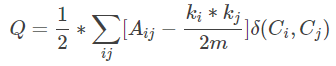模块度值的大小主要取决于网络中结点的社区分配C，即网络的社区划分情况，可以用来定量的衡量网络社区划分质量，其值越接近1，表示网络划分出的社区结构的强度越强，也就是划分质量越好。因此可以通过最大化模块度Q来获得最优的网络社区划分。

Python：可以直接使用Community.modularity()包计算模块度。

若已知样本的真实类别标签labelstruelabelstrue ,和聚类算法得到的标签labelspredlabelspred，ARI是计算两种标签分布相似性的函数，该函数对标签的定义形式没有要求。ARI定义如下：

如果C是真实类别，K是聚类结果，我们定义a和b分别是：

a: 在C和K中都是同一类别的样本对数

b: 在C和K中都是不同类别的样本对数

Raw Rand Index公式如下：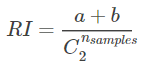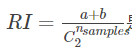是样本所有的可能组合对.

RI不能保证在类别标签是随机分配的情况下，其值接近0（极端情况是类别数和样本数相等）.为了解决这个问题，ARI被提出，它具有更高的区分度.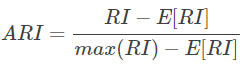### 三、标准化互信息NMI（Normalized Mutual Information）

假设对于N个样本点的两种标签划分为U 和 V. 熵为划分集的不准确性，定义如下: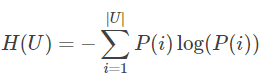其中 P(i)=|Ui|/NP(i)=|Ui|/N表示任取一个样本划分为 UiUi的概率. 对于V同时成立: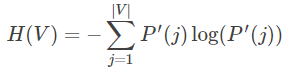其中 P′(j)=|Vj|/NP′(j)=|Vj|/N. U和V之间的互信息(MI) 可以通过下式进行计算: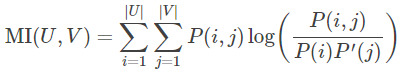其中 P(i,j)=|Ui∩Vj|/NP(i,j)=|Ui∩Vj|/N表示两个样本点划分相同的类 Ui和VjUi和Vj的概率.
也可以通过集合的势来表示: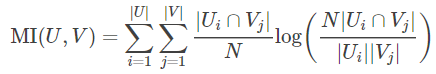规则化互信息定义如下：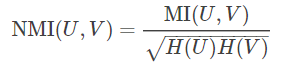This value of the mutual information and also the normalized variant is not adjusted for chance and will tend to increase as the number of different labels (clusters) increases, regardless of the actual amount of “mutual information” between the label assignments.
The expected value for the mutual information can be calculated using the following equation, from Vinh, Epps, and Bailey, (2009). In this equation, ai=|Ui|ai=|Ui| (the number of elements in UiUi) and bj=|Vj|bj=|Vj|(the number of elements in VjVj).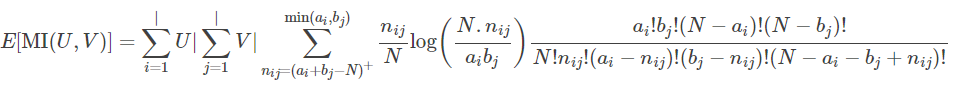Using the expected value, the adjusted mutual information can then be calculated using a similar form to that of the adjusted Rand index: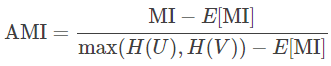Python: 使用sklearn.metrics.normalized_mutual_info_score(labels_true, labels_pred)计算。

展开全文• 复杂网路中，LFR复杂网络生成图代码，以及NMI代码，内附生成图与详解，仅供个人使用
• NMI 评价方法（matlab），用于评价分类和聚类的FUNCTION，输入是正确的类标和试验后的类标
• 计算重叠互信息NMI源码 linux环境 参考文献：Lancichinetti A Fortunato S Kertész J Detecting the overlapping and hierarchical community structure in complex networks[J] New Journal of Physics 2009 11 3 ...
• 基于脉冲耦合神经网络的图像NMI特征提取及检索方法，论文的程序仿真，带单处理和批量处理功能，里面包含原论文，可以用于场景相似性检测，以图识图等，matlab仿真实现
• Kazerounian博士认为物联网将推动其他智能联网设备的发展，未来人们只需使用一个移动设备，比如智能手机或平板电脑，就可直接监控其他与物联网端点相连的其他系统，比如汽车、能源、医疗保健等
• Shafer，用于不断增长的神经网络的互信息的多元扩展，神经网络（2017 年），印刷中。 依赖项：Octave/MATLAB 版本：NONE R 版本：pracma（必需）、编译器（可选） nmi.m 是一个 Octave/MATLAB 函数，用于计算二进制...
• 代码 def nmi(X, Y): """ X:n*Kx Y:n*Ky """ X = X.T Y = Y.T def cmp(x, y): """a b c d""" a = (1 - x).dot(1 - y) d = x.dot(y) c = (1 - y).dot(x) b = (1 - x).dot(y) return a, b, c, d def h(w, n): """h(w,n...

# 参考文献：

Normalized Mutual Information to evaluate overlapping community finding algorithms

# 1.互信息

,代表了社区矩阵，他们的维度分别为
n代表节点数，Kx和Ky代表社区数
代表第m个节点隶属于第i个社区的情况
我们先比较两个社区，定义a,b,c,d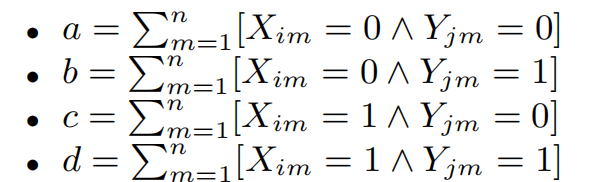若 a+d=n，则b=c=0，所以两个向量完全相同
这两个向量的互信息如下所示：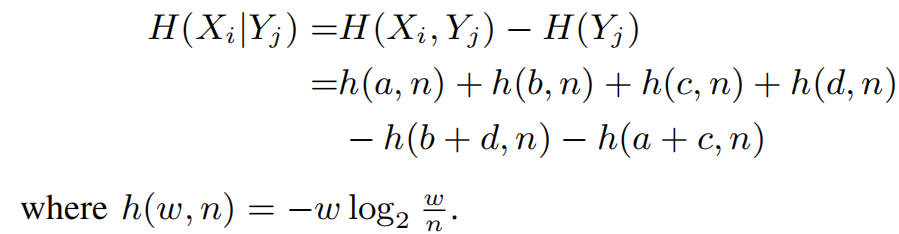如果两个向量互补的话那互信息也会很高，我们要限制这样的情况：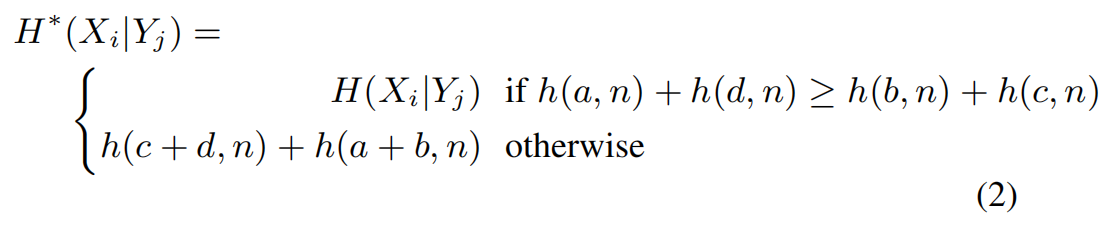我们将Xi和Y去计算，肯定找最相似的那一个社区，因此找最小的，原理：当两个社区完全重叠，他们的H为0。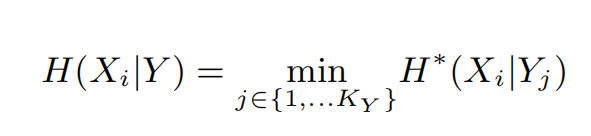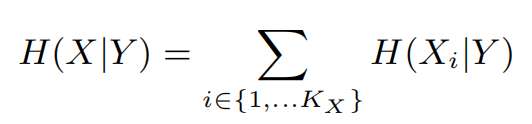计算X自身的信息熵：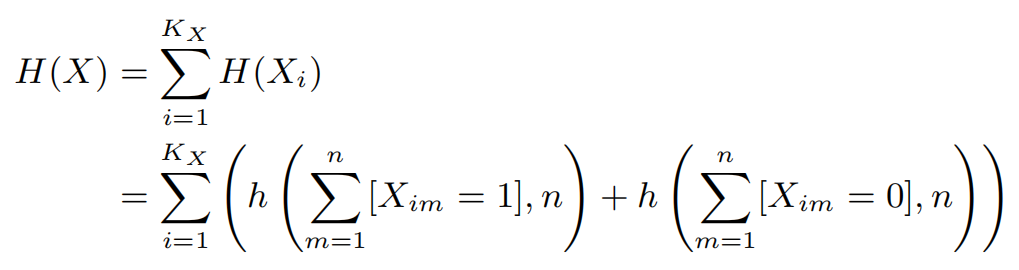# 2.重要的恒等式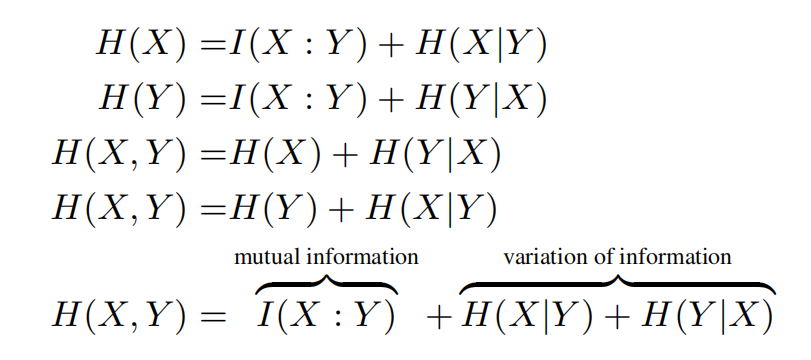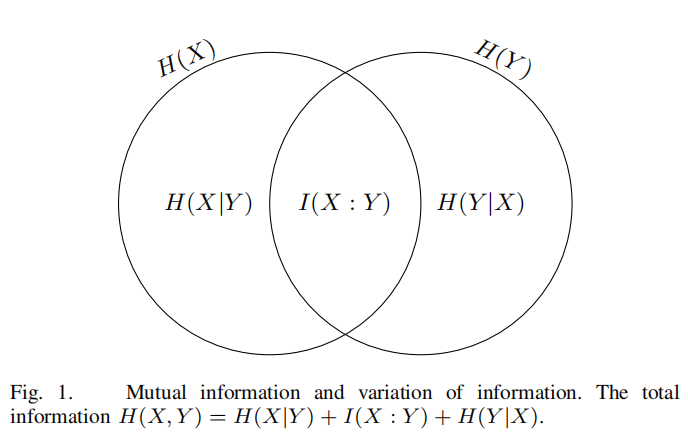平均互信息，因为近似有偏差：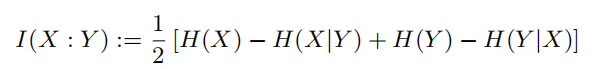# 3.归一化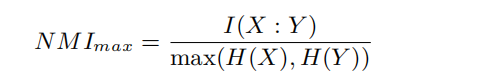# 4.效果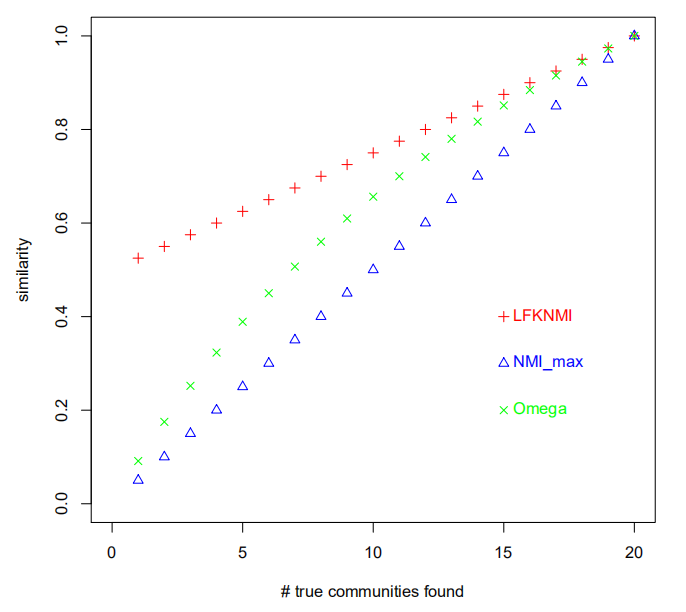可以看出当检测出来的社区越少得分越低，社区越多得分越高且线性相关，明显好于其他两个。

# 6.代码

def nmi(X, Y):
"""
X:n*Kx
Y:n*Ky
"""
X = X.T
Y = Y.T
def cmp(x, y):
"""a b c d"""
a = (1 - x).dot(1 - y)
d = x.dot(y)
c = (1 - y).dot(x)
b = (1 - x).dot(y)
return a, b, c, d

def h(w, n):
"""h(w,n)"""
if w == 0:
return 0
else:
return -w * np.log2(w / n)

def H(x, y):
"""H*(Xi|Yj)"""
a, b, c, d = cmp(x, y)
n = len(x)
if h(a, n) + h(d, n) >= h(b, n) + h(c, n):
return h(a, n) + h(b, n) + h(c, n) + h(d, n) - h(b + d, n) - h(a + c, n)
else:
return h(c + d, n) + h(a + b, n)
def H_uncond(X):
"""H(X)"""
return sum(h(x.sum(), len(x)) + h(len(x) - x.sum(), len(x)) for x in X)

def H_cond(X, Y):
"""H(X|Y)"""
m, n = X.shape, Y.shape
scores = np.zeros([m, n])
for i in range(m):
for j in range(n):
scores[i, j] = H(X[i], Y[j])
return scores.min(axis=1).sum()

H_X = H_uncond(X)
H_Y = H_uncond(Y)

"""I(X:Y)"""
I_XY = 0.5 * (H_X + H_Y - H_cond(X, Y) - H_cond(Y, X))

"""NMImax"""
return I_XY / max(H_X, H_Y)

展开全文深度学习
• 针对传统社交网络社区划分算法普遍缺乏对节点属性、链接属性的综合考虑和充分表达利用节点与链接属性信息的模型和机制等问题, 提出了一种融合节点与链接属性的社交网络社区划分算法。该算法融合节点属性的相似度、...社区划分
• 在线社交平台产生大量可建模为属性网络的数据，SNE（social network embedding）表示...在五个真实属性网络上的实验结果表明，相比SNE，ANESC学到的表示在聚类任务上NMI值提高5%~11%，在分类任务上准确率提高0.3%~7%。
• 2004年Newman等人提出了模块度Q，之后广泛应用于衡量社区的划分质量，下面公式适用于无向无权的同质网络。若社区内节点的边数越多，Q值越大，相反，Q值越小。 Q=12m∑i,j[Aij−didj2m]δ(Ci,Cj) Q=\frac{1}{2m}\sum_...

## 社区发现评价指标Q和NMI讲解、代码实现

### 模块度Q

2004年Newman等人提出了模块度Q，之后广泛应用于衡量社区的划分质量，下面公式适用于无向无权的同质网络。若社区内节点的边数越多，Q值越大，相反，Q值越小。
Q = 1 2 m ∑ i , j [ A i j − d i d j 2 m ] δ ( C i , C j ) Q=\frac{1}{2m}\sum_{i,j}\Big[A_{ij}-\frac{d_id_j}{2m}\Big]\delta(C_i,C_j)

m 为 网 络 中 的 边 数 ； A i j 为 邻 接 矩 阵 A 中 的 元 素 ， m为网络中的边数；A_{ij}为邻接矩阵A中的元素，

若 节 点 i , j 相 连 ， 则 A i j = 1 , 否 则 A i j = 0 ; 若节点i,j相连，则A_{ij}=1,否则A_{ij}=0;

C i 为 节 点 i 所 属 的 社 区 ， C j 为 节 点 j 所 属 的 社 区 ； C_i为节点i所属的社区，C_j为节点j所属的社区；

当 i , j 处 于 同 一 社 区 时 ， δ ( C i , C j ) = 1 ， 否 则 为 0 ； 当i,j处于同一社区时，\delta(C_i,C_j)=1，否则为0；

d i 代 表 节 点 i 的 度 ； d_i代表节点i的度；

d i d j 2 m 表 示 在 随 机 网 络 中 节 点 i 和 节 点 j 之 间 存 在 边 的 可 能 性 。 \frac{d_id_j}{2m}表示在随机网络中节点i和节点j之间存在边的可能性。

模块度Q也可表示为
Q = ∑ c = 1 k [ l c m − ( d c 2 m ) 2 ] Q=\sum_{c=1}^{k}\Big[\frac{l_c}{m}-(\frac{d_c}{2m})^2\Big]

上 式 中 ， m 为 网 络 中 的 总 边 数 ； k 为 社 区 个 数 ， 上式中，m为网络中的总边数；k为社区个数，

l c 为 社 区 c 内 部 的 连 接 边 数 ， d c 为 社 区 c 内 所 有 节 点 的 度 之 和 。 l_c为社区c内部的连接边数，d_c为社区c内所有节点的度之和。

其中，Q的取值范围为[-0.5, 1]

注：模块度Q适用于已知真实社区或未知真实社区划分结果的评估

### 标准化互信息NMI

NMInormalized mutual information标准化互信息，也称为归一化互信息，是目前广泛使用的一种社区划分评价指标，用于度量算法所得到的社区和真实社区划分之间的相似程度，也用于评估聚类结果的相似程度。
N M I ( R , F ) = − 2 ∑ i = 1 C R ∑ j = 1 C F N i j l o g ( N i j S N i ∗ N ∗ j ) ∑ C R N i ∗ l o g ( N i ∗ / S ) + ∑ C F N ∗ j l o g ( N ∗ j / S ) NMI(R,F)=\frac{-2\sum_{i=1}^{C_R}\sum_{j=1}^{C_F}N_{ij}log(\frac{N_{ij}S}{N_{i*}N_{*j}})}{\sum^{C_R}N_{i*}log(N_{i*}/S)+\sum^{C_F}N_{*j}log(N_{*j}/S)}

其 中 ， 矩 阵 N 中 的 行 表 示 真 实 的 社 区 ， 列 表 示 算 法 得 到 的 社 区 ； 其中，矩阵N中的行表示真实的社区，列表示算法得到的社区；

矩 阵 中 第 i 行 的 元 素 表 示 为 N i ∗ , 第 j 列 的 元 素 表 示 为 N ∗ j ; 矩阵中第i行的元素表示为N_{i*},第j列的元素表示为N_{*j};

N i j 表 示 真 实 社 区 与 算 法 所 得 到 的 社 区 相 同 的 节 点 个 数 ； S 为 节 点 个 数 ； N_{ij}表示真实社区与算法所得到的社区相同的节点个数；S为节点个数；

C R 表 示 真 实 社 区 个 数 ， C F 表 示 算 法 所 得 到 的 的 社 区 个 数 。 C_R表示真实社区个数，C_F表示算法所得到的的社区个数。

当 算 法 所 得 到 的 的 社 区 划 分 与 网 络 中 的 真 实 社 区 划 分 完 全 一 致 时 ， N M I = 1 ; 当算法所得到的的社区划分与网络中的真实社区划分完全一致时，NMI=1;

当 算 法 所 得 到 的 划 分 社 区 与 网 络 中 的 真 实 社 区 划 分 完 全 独 立 时 ， N M I = 0 。 当算法所得到的划分社区与网络中的真实社区划分完全独立时，NMI=0。

显然，NMI的取值范围为[0,1]

注：NMI适用于已知真实社区结构的数据集上的社区划分评估

### 代码实现

🎈

import networkx as nx
import math

class Evaluate:
"""
社区划分结果评估
"""

def __init__(self, G, cur_community, real_community):
"""
评价指标初始化
:param G: 图
:param cur_community: 当前社区划分结果 {id： nodes}
:param real_community: 真实社区结果{id : nodes}
:return: null
"""
self.G = G
self.cur_community = cur_community
self.real_community = real_community

def Q(self):
"""
计算划分社区的模块度Q(modularity)
未知和已知社区的评估指标
:return: Q_value
"""
nodes_number = self.G.number_of_nodes()
edges_number = self.G.number_of_edges()
Q_value = 0
for key in self.cur_community.keys():
#社区c
c = self.cur_community[key]
#社区内结点度数之和
degrees = 0
#社区内部边的总数
inter_edges = 0
for u in c:
u_neighbors = set(nx.all_neighbors(self.G, u))
degrees += len(u_neighbors)
for nbr in u_neighbors:
if nbr in c:
inter_edges += 1
#因为是无向图，所以边数要除以2
inter_edges /= 2
#cq = inter_edges / edges_number - math.pow(degrees / (2 * edges_number), 2)
cq = inter_edges / edges_number - (degrees / (2 * edges_number)) ** 2
Q_value += cq
return Q_value

def NMI(self):
"""
标准化互信息值( Normalized mutual information)
已知真实社区划分结果的评价指标
行代表真实社区划分（real_community）
列代表当前社区划分结果(cur_community)
:return:NMI_value
"""
nodes_number = self.G.number_of_nodes()
r_id = [key for key in self.real_community]
c_id = [key for key in self.cur_community]
#分子
sum_rc = 0
#分母两项之和
sum_r = sum_c = 0

for i in range(0, len(r_id)):
temp = 0
nodes_i = set(self.real_community[r_id[i]])
for j in range(0, len(c_id)):
nodes_j = set(self.cur_community[c_id[j]])
common = nodes_i & nodes_j
a = len(common) * nodes_number / (len(nodes_i) * len(nodes_j))
if a > 0:
temp += len(common) * math.log10(a)
sum_rc += temp

for i in range(0, len(r_id)):
nodes_i = set(self.real_community[r_id[i]])
sum_r += len(nodes_i) * math.log10(len(nodes_i) / nodes_number)
for j in range(0, len(c_id)):
nodes_j = set(self.cur_community[c_id[j]])
sum_c += len(nodes_j) * math.log10(len(nodes_j) / nodes_number)

sum_rc = sum_rc * (-2)
NMI_value = sum_rc / (sum_r + sum_c)
return NMI_value


### 参考文献

Newman M E J,Girvan M. Finding and evaluating community structure in networks.[J]. Physical review. E, Statistical, nonlinear, and soft matter physics,2004,69(2 Pt 2).

 赵卫绩,张凤斌,刘井莲.复杂网络社区发现研究进展[J].计算机科学,2020,47(02):10-20.

本人理解尚浅，若有错误之处欢迎大家指出！

展开全文社区发现
• NMI(Normalized Mutual Information)常用在聚类中，度量两个聚类结果的相近程度。是社区发现(community detection)的重要衡量指标，基本可以比较客观地评价出一个社区划分与标准划分之间相比的准确度。NMI的值域是0...数据挖掘
• 机器学习的模型随机打印标签，ACC和NMI计算方法？ 机器学习中实现了一个简单的神经网络，但发现在验证的时候，模型对数据打的标签很随意，比如一个四分类问题，groundtruth（简称gt）是gt = [0,0,0,1,2,3,3]，而...机器学习 聚类分类 机器学习 adaboost算法
• 本资源收录了关于多层网络的模块度最大化问题的（multiplex modularity maximization，mmm）genlouvain贪婪算法，pmm算法与自编谱方法，并收录了一些常用的多层网络数据（包括实际数据与合成数据），并附上了算法在...
• cp /proc/sys/kernel/watchdog_thresh /proc/sys/kernel/watchdog_thresh.template echo 30 > /proc/sys/kernel/watchdog_thresh echo "kernel.watchdog_thresh=30" >> /etc/sysctl.conf ...
• 鉴于计算代价高昂的谱聚类无法满足海量网络社区发现的... 实验结果表明, 与代表性算法(CPM, Link, COPRA, SSDE) 相比较, SCEA 能够挖掘出具有更高规范化互信息(NMI) 的网络重叠社区结构, 且具有相对较好的鲁棒性.</p>
• Face the past with the least regrets, face the present with the least waste and face the future with the ...社团划分结果评估指标：Q、ARI、NMI一、模块度Q（Modularity）模块度也称模块化度量值，是目前常用
• 系统或者网络占用过多CPU，造成内核软死锁（soft lockup）。Soft lockup名称解释：所谓，soft lockup就是说，这个bug没有让系统彻底死机，但是若干个进程（或者kernel thread）被锁死在了某个状态（一般在内核区域...
• 一、已知真实社区划分结果 ...1.NMI指数，互信息和标准化互信息 具体公式和matlab代码参见博客，Python代码参加，C++代码参见 function MIhat = nmi( A, B ) %NMI Normalized mutual in...
• 前言：最近刚定下的课题，现在主要学习网络基础概念的知识，凡是学习总是得做下总结笔记才能比较清楚。也分享给大家一起学习吧，如有错误可以提出私信我或者评论。 社会网络通常显示出较强的社区效应，网络中的节点...机器学习
• 本文主要讲解如何重启RHEL 8或者CentOS 8网络以及如何解决RHEL8和CentOS8系统的网络管理服务报错，当我们安装好RHEL 8或者 CentOS 8，重启启动网络时，会出现以下报错： # systemctl restart network.service 报错...
• 一、已知真实社区划分结果1.NMI指数，互信息和标准化互信息function MIhat = nmi( A, B )%NMI Normalized mutual information% ...
• ## 附源码|复杂网络社区发现--GN算法

千次阅读 多人点赞 2020-06-14 23:20:23
由Girvan和Newman提出的GN算法在近几年已成为社团结构分析的一种标准算法，他的基本思想是从网络的整体出发，不断地从网络中移除介数最大的边，从而获得最佳的社团结构。边介数定义为网络中经过每条边的最短路径的...python 社区发现
• 精确度：精度以输出群集和参考群集之间的标准化互信息（NMI）进行衡量。基准网络由5000个节点组成，社区规模在20到200之间。 分层精度：该图显示了该算法很好地揭示了不同级别的三角网络中节点的层次结构...聚类 python
• 历时半个多月，我们终于从刚开始的张量学习 ，到现在的张量网络，本人真的是一路艰辛，哎 ，打油诗一首表心意： 本是计科专业狗，年少无知四顾首。 无赖量子吸引我，前路茫茫无处走。 早知人生本就难，科研门前抖一...矩阵 量子机器学习...